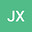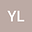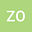The existence and non-existence of ground state solutions to Schrödinger systems with general potentials
•••Jia-Lin Xu
Southwest University

Corresponding Author:[email protected]

Author ProfileZeng-Qi Ou
Southwest University
Author Profile## Abstract

In this paper, we first study the existence of ground state solutions for the following Schrödinger systems { − ∆ u + V ∞ u = G u ( u , v ) , x ∈ R N , − ∆ v + V ∞ v = G v ( u , v ) , x ∈ R N , u , v > 0 , u , v ∈ H 1 ( R N ) , where N≥3 and G ∈ C 2 ( ( R + ) 2 , R ) . And then, by using variational method and projections on Nehari-Poho z ̆ aev type manifold, we will prove the nonexistence of ground state solutions for the coupled Schrödinger systems { − ∆ u + V ( x ) u = G u ( u , v ) , x ∈ R N , − ∆ v + V ( x ) v = G v ( u , v ) , x ∈ R N , u , v > 0 , u , v ∈ H 1 ( R N ) .